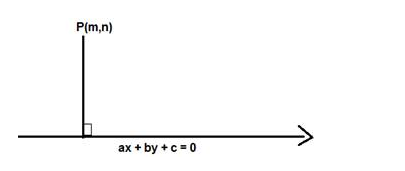# Find the values of k for which the length`
Question:

Find the values of k for which the length of the perpendicular from the point (4, 1) on the line 3x – 4y + k = 0 is 2 units

Solution:

Given: Point (4,1) , line 3x – 4y + k = 0 and length of perpendicular is 2 units

To find: The values of k

Formula used:

We know that the length of the perpendicular from (m,n) to the line ax + by + c = 0 is given by,

$D=\frac{|a m+b n+c|}{\sqrt{a^{2}+b^{2}}}$The equation of the line is $3 x-4 y+k=0$

Here $m=4$ and $n=1, a=3, b=-4, c=k$ and $D=2$ units

$D=\frac{|3(4)-4(1)+k|}{\sqrt{3^{2}+4^{2}}}=2$

$D=\frac{|12-4+k|}{\sqrt{9+16}}=\frac{|8+k|}{\sqrt{25}}=\frac{|8+k|}{5}=2$

$|8+\mathrm{k}|=2 \times 5=10$

$8+\mathrm{k}=10$. or $8+\mathrm{k}=-10$

$\mathrm{k}=10-8$ or $\mathrm{k}=-10-8$

$\mathrm{k}=2$ or $\mathrm{k}=-18$

The values of $k$ are 2 and $-18$